Question

# If the original ice added to the water was already 0Celcius and some of it was...

If the original ice added to the water was already 0Celcius and some of it was wet, what effect would this have had on your calculated value of Lf?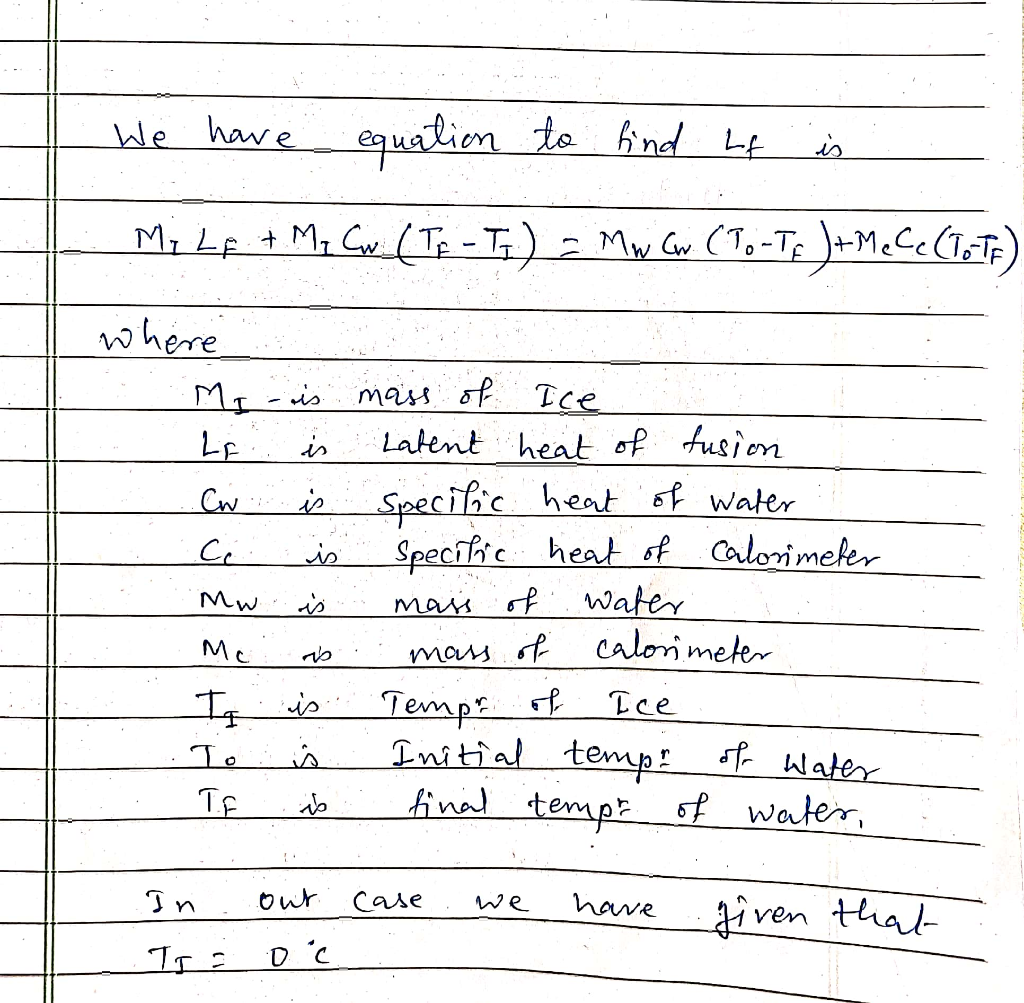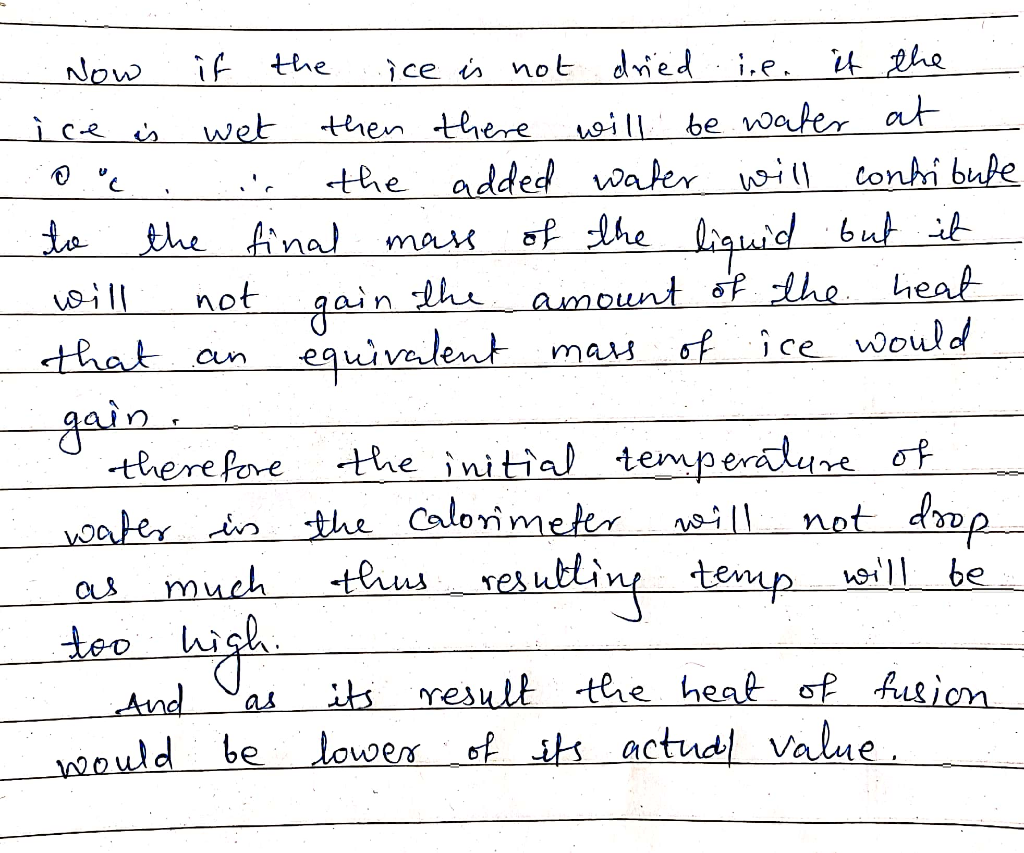#### Earn Coins

Coins can be redeemed for fabulous gifts.

Similar Homework Help Questions
• ### Of ice at -S °C is added to 40 kg of water initially at 20 °C. Assume that the container is well ...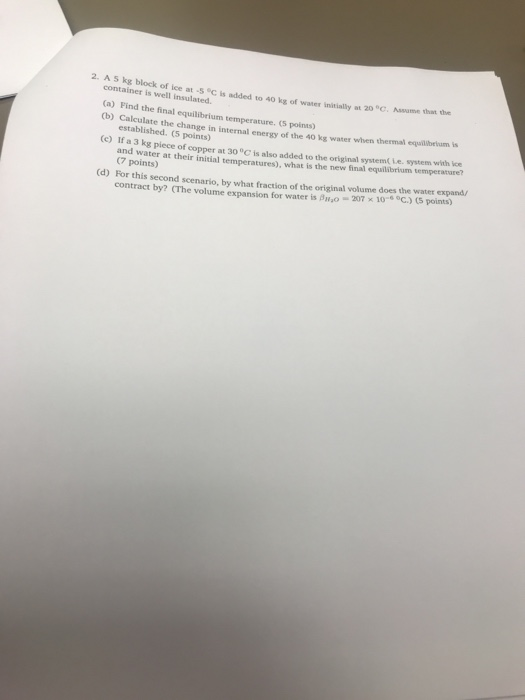of ice at -S °C is added to 40 kg of water initially at 20 °C. Assume that the container is well insulated. (a) Find the final equilibrium temperature. (5 points) 0b) Caleulate the change in internal energy of the 40 kg water when thermal equilberium is established. (5 points) (c) If a 3 kg piece of copper at 30°C is also added to the original system( Le. system with ice d wat an (7 points) er at their initial...

• ### (TCO 7) Heat is added to a 5.0 kg piece of ice at a rate of...

(TCO 7) Heat is added to a 5.0 kg piece of ice at a rate of 454.0 kW. How long will it take for the ice at 0.0 °C to melt? (For water, Lf = 334 kJ/kg and Lv = 2257 kJ/kg.)

• ### Why is already-heated water added to the water bath under the reaction mixture as opposed to...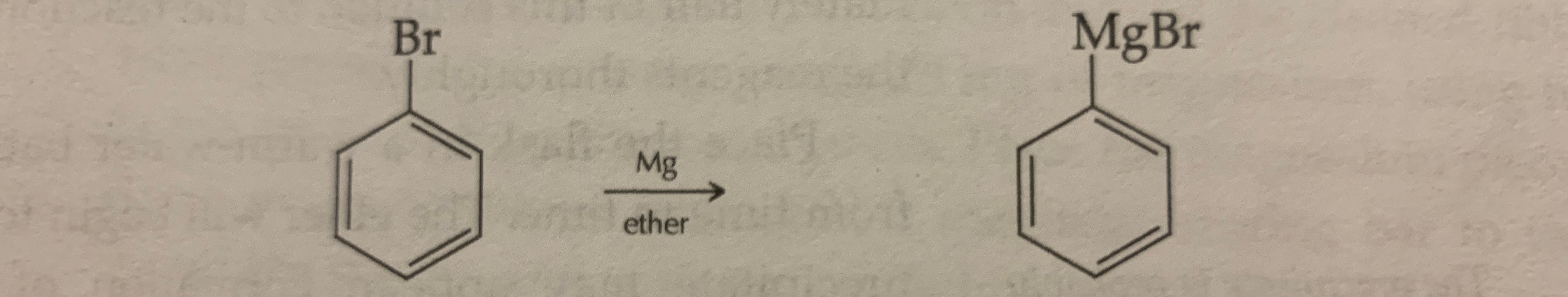Why is already-heated water added to the water bath under the reaction mixture as opposed to simply heating the water directly with a hot plate during the Grignard reaction? Why is the initial portion of bromobenzene in ether added before additional ether is added? Why is additional ether added before the second portion of bromobenzene is added? During what part of the purification procedure is any unreacted acetophenone removed from the product? Be specific. Answer the question assuming that there...

• ### How much ice (at 0°C) must be added to 1.05 kg of water at 87 °C...

How much ice (at 0°C) must be added to 1.05 kg of water at 87 °C so as to end up with all liquid at 20 °C? (ci = 2000 J/(kg.°C), cw = 4186 J/(kg.°C), Lf=3.35×1053.35×105 J/kg, Lv=2.26×1062.26×106 J/kg)

• ### A piece of ice at 0 °C and weighting 41 g is added to a beaker...

A piece of ice at 0 °C and weighting 41 g is added to a beaker of water at 16.0 °C. All the ice melts, and the temperature of the water in the beaker decreases to 8.0 °C. What was the original volume of water was in the beaker? (i.e., the initial volume of water in the beaker) Density of water = 1.0 /mL

• ### When 15-kg of some unknown at 180°C is added to 4-kg of water at 20°C the...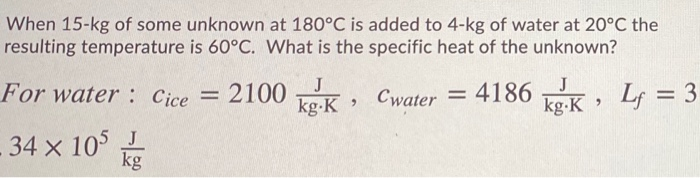When 15-kg of some unknown at 180°C is added to 4-kg of water at 20°C the resulting temperature is 60°C. What is the specific heat of the unknown? J Cwater = 4186 kg:K, Lf = 3 For water : Cice = 2100 kg.k - 34 x 105 kg

• ### Suppose you put some water in your ice-cube tray and then place the tray in the...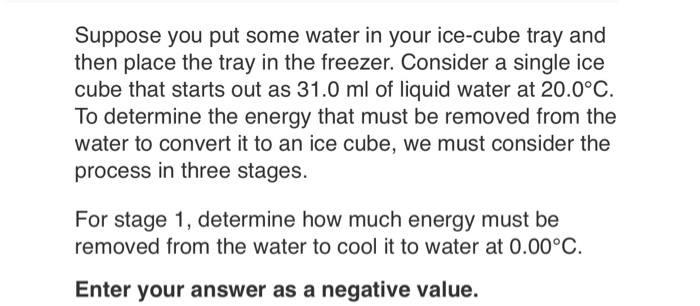Suppose you put some water in your ice-cube tray and then place the tray in the freezer. Consider a single ice cube that starts out as 31.0 ml of liquid water at 20.0°C. To determine the energy that must be removed from the water to convert it to an ice cube, we must consider the process in three stages. For stage 1, determine how much energy must be removed from the water to cool it to water at 0.00°C Enter...

• ### If heat is added to ice and liquid water in a closed container and, after the...

If heat is added to ice and liquid water in a closed container and, after the addition of the heat, ice and liquid water remain, a. the vapor pressure of the water will decrease. b. the temperature will increase somewhat. c. the temperature will decrease somewhat. d. the vapor pressure of the water will rise. e. the vapor pressure of the water will remain constant.

• ### A. A 32.1 g of ice at 0 C is added to 120g of water in...

A. A 32.1 g of ice at 0 C is added to 120g of water in a 64.8 g aluminum cup. the cup and the water have an initial temperature of 23.7 C. Calculate the equilibrium temperature of the cup and its contents. B. Calculate the equilibrium temperature of the cup and its contents if the aluminum cup is replaced with one of equal mass made from glass.

• ### Thank you! A ketone is added to acidic water (i.e., water that has some HCl added to it), and, to some extent, a hydrate is formed. What are the mechanistic steps for this reaction? To answer, use...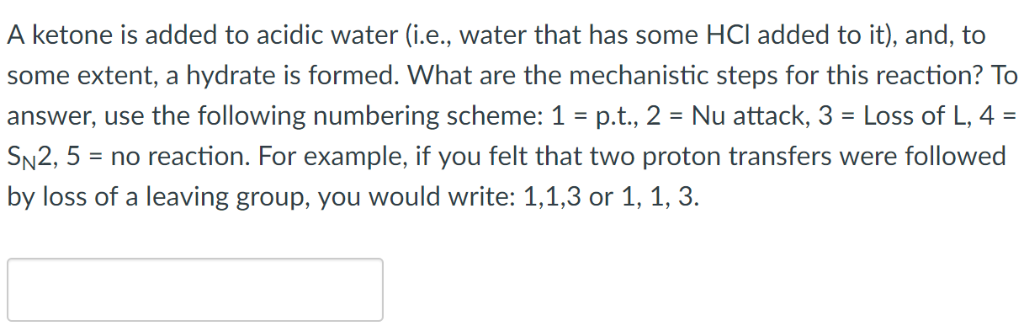Thank you! A ketone is added to acidic water (i.e., water that has some HCl added to it), and, to some extent, a hydrate is formed. What are the mechanistic steps for this reaction? To answer, use the following numbering scheme: 1-pt., 2 Nu attack, 3-Loss of L, 4 = SN2, 5-no reaction. For example, if you felt that two proton transfers were followed by loss of a leaving group, you would write: 1,1,3 or 1, 1, 3 A secondary...